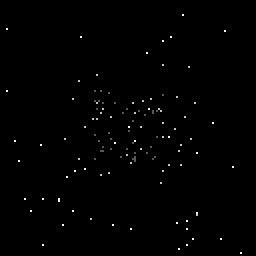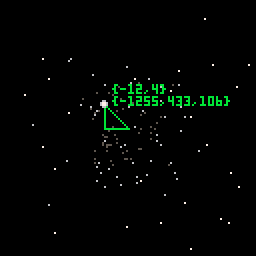# Teaching your teen to code a starfield in PICO-8

Kids love starfields. It’s a perfect coding session with a nice visual result and basic highschool math. We’re going to build this:When coding with kids, always keep it simple and at their level. I know most of you reading this are math wizards but kids tend not to be super impressed by your vector math and matrix calculations (believe me, I’ve tried). You’re going for quick results and simple code, on their math level. If you push them too hard you’ll loose them to another Overwatch session. We’re trying to avoid that.

A star object is a 3D vector, that’s math for a basic x,y,z coordinate in space. [1,2,3] would be a star at x=1, y=2, z=3 in space. We will have a bunch of star objects with random x,y,z coordinates. Since we want them to move towards us we just decrease the z coordinate for each star every frame, keeping their x and y coordinate intact. In order to get it to look like a starfield we simply calculate the x and y screen coordinate by dividing the star x and y coordinate with z. Big z will give us small x and y screen coordinates. Small Z will give us the opposite. We’ll keep the x and y coordinates for a star in the range -2500 to 2500 and the z in the range 0 to 150 A quick and dirty perspective divide. That’s all the math. It looks like this.You can see the x,y,z star coordinates for the star below the star screen coordinates which are just x and y divided by z.

Let’s start by adding the following code in the code tab.

``````-- this is called once when the program starts
function _init()
-- calls our starfield init code and sets up all the stars
sf.generate()
end

-- normal pico8 frame rate is 30
-- add this function to get 60 fps
function _update60()
end

-- called every frame
function _draw()
-- clear screen with black
cls(0)
-- update the starfield
sf.update()
end
``````

We’ll put the starfield code in another pico-8 code tab. You can copy and pase the code below.

``````sf={}
sf.stars={}
-- let's have 128 stars
sf.starcount=128
-- max z for a star is 150
sf.maxd=150

sf.generate=function()
local range=2500
--create table with stars
for i=1,sf.starcount do
--keep x any y in ranmge -2500 to 2500
xp=flr(range-rnd(range*2))
yp=flr(range-rnd(range*2))
zp=rnd(sf.maxd)
end
end

sf.update=function()
for i=1,#sf.stars do
sf.stars[i].z=sf.stars[i].z-1
if sf.stars[i].z<=0 then
sf.stars[i].z=sf.maxd
end
end
--iterate all the stars
for i=1,#sf.stars do
--calc star pos xp=x/z yp=y/z
local cz=sf.stars[i].z
local cx=sf.stars[i].x/cz
local cy=sf.stars[i].y/cz
--if star is outside sceen
--set z position to max dist
if cx<-64 or cx>64 then
sf.stars[i].z=sf.maxd
end
if cy<-64 or cy>64 then
sf.stars[i].z=sf.maxd
end
--set the color of the star
local cols={7,6,5}
local ci=1+flr(cz/sf.maxd*#cols)
--plot the star
pset(64+cx,64+cy,cols[ci])
end
end
``````

And that’s it. A star field project in PICO-8. It’s a two hour session for a 14 year old including setting up pico-8, explaining basic Lua and writing the project.

Source code and cartridge is available here https://www.lexaloffle.com/bbs/?tid=33840

PICO-8 is available from here https://www.lexaloffle.com/pico-8.php